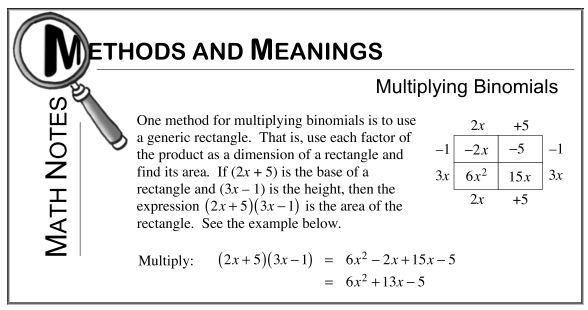### Home > GC > Chapter 2 > Lesson 2.2.2 > Problem2-70

2-70.

Review how to multiply binomials by reading the Math Notes box for this lesson. Then rewrite each of the expressions below by multiplying binomials and simplifying the resulting expression.

1. $(4x + 1)(2x-7)$

1. $(5x-2)(2x + 7)$

1. $(4x − 3)(x-11)$

1. $(−3x + 1)(2x-5)$Review the method for multiplying binomials by examining the Math Notes box at left.

Draw a rectangle with its width and length each divided once.

Label the rectangle's length with the first binomial, with one term corresponding to each half.

$\left. \begin{array} { | c | c | c | } \hline x & { } & { + 1 } \\ \hline - 7 & { 28 x } & { - 7 } \\ \hline 2 x & { 8 x ^ { 2 } } & { } \\ \hline \end{array} \right.$

Do the same for the width and the second binomial.

For each of the four portions of the rectangle, find the product of the term on its width and the term on its length.

$8x^2 − 26x − 7$

Follow the same steps for parts (b) through (d).